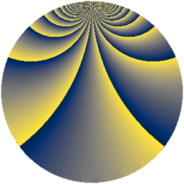# Properties

 Label 361.2.gLevel $361$ Weight $2$ Character orbit 361.g Rep. character $\chi_{361}(20,\cdot)$ Character field $\Q(\zeta_{19})$ Dimension $540$ Newform subspaces $1$ Sturm bound $63$ Trace bound $0$

# Related objects

## Defining parameters

 Level: $$N$$ $$=$$ $$361 = 19^{2}$$ Weight: $$k$$ $$=$$ $$2$$ Character orbit: $$[\chi]$$ $$=$$ 361.g (of order $$19$$ and degree $$18$$) Character conductor: $$\operatorname{cond}(\chi)$$ $$=$$ $$361$$ Character field: $$\Q(\zeta_{19})$$ Newform subspaces: $$1$$ Sturm bound: $$63$$ Trace bound: $$0$$

## Dimensions

The following table gives the dimensions of various subspaces of $$M_{2}(361, [\chi])$$.

Total New Old
Modular forms 576 576 0
Cusp forms 540 540 0
Eisenstein series 36 36 0

## Trace form

 $$540 q - 16 q^{2} - 32 q^{3} - 40 q^{4} - 17 q^{5} - 7 q^{6} - 11 q^{7} - 4 q^{8} - 94 q^{9} + O(q^{10})$$ $$540 q - 16 q^{2} - 32 q^{3} - 40 q^{4} - 17 q^{5} - 7 q^{6} - 11 q^{7} - 4 q^{8} - 94 q^{9} - 58 q^{10} - 11 q^{11} + 5 q^{12} - q^{13} - 33 q^{14} + 11 q^{15} - 22 q^{16} - 56 q^{17} + 20 q^{18} - q^{19} + 25 q^{20} + 11 q^{21} - 116 q^{22} + q^{23} + 33 q^{24} - 23 q^{25} - 80 q^{26} - 2 q^{27} + 12 q^{28} - 33 q^{29} - 69 q^{30} + 17 q^{31} + 44 q^{32} - 3 q^{33} + 35 q^{34} + 27 q^{35} + 36 q^{36} + 17 q^{37} + 19 q^{38} - 53 q^{39} + 71 q^{40} + 29 q^{41} + 71 q^{42} - 169 q^{43} + 59 q^{44} - 44 q^{45} + 15 q^{46} - 32 q^{47} - 96 q^{48} - 67 q^{49} - 2 q^{50} - 29 q^{51} + 71 q^{52} + 23 q^{53} - 350 q^{54} + 35 q^{55} - 241 q^{56} + 59 q^{57} + 67 q^{58} + 47 q^{59} + 99 q^{60} + 39 q^{61} + 52 q^{62} - 20 q^{63} + 80 q^{64} + q^{65} + 113 q^{66} + 53 q^{67} + 72 q^{68} - 246 q^{69} - 27 q^{70} + 47 q^{71} + 176 q^{72} + 53 q^{73} - 107 q^{74} - 115 q^{75} - 245 q^{76} + 69 q^{77} - 117 q^{78} + 53 q^{79} - 205 q^{80} - 54 q^{81} + 21 q^{82} + 26 q^{83} + 152 q^{84} + 83 q^{85} - 267 q^{86} + 8 q^{87} + 161 q^{88} - 55 q^{89} - 127 q^{90} + 51 q^{91} + 133 q^{92} + 93 q^{93} - 141 q^{94} - 22 q^{95} - 19 q^{96} + 14 q^{97} + 57 q^{98} + 94 q^{99} + O(q^{100})$$

## Decomposition of $$S_{2}^{\mathrm{new}}(361, [\chi])$$ into newform subspaces

Label Dim $A$ Field CM Traces $q$-expansion
$a_{2}$ $a_{3}$ $a_{5}$ $a_{7}$
361.2.g.a $540$ $2.883$ None $$-16$$ $$-32$$ $$-17$$ $$-11$$